# C# 数据类型转换 显式转型、隐式转型、强制转型

C# 的类型转换有 显式转型隐式转型 两种方式。

不同类型的数据进行操作(加减乘除赋值等等)，是需要进行 类型转换 后，才能继续操作。所以需要“类型转换”。

```        int i = 66666;
long b = i;　　　　//转为 long 类型后为 b 赋值
```

通常情况下，多种 值类型 进行计算时，系统会自动进行隐式转型，并且总是转为范围更大的数据类型，并且不会发生精确度变化、数字大小变化等等。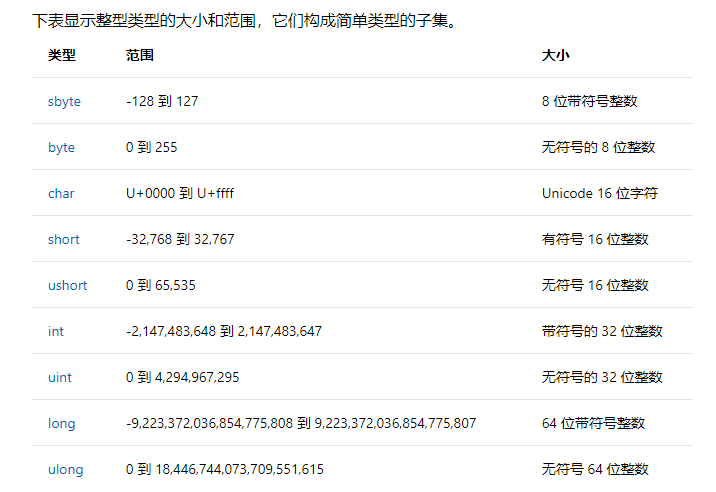char 是整型！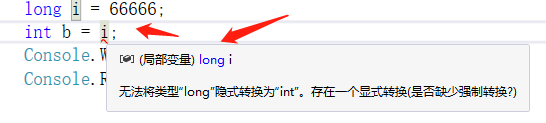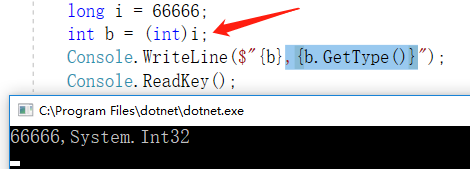不应将范围大的类型 往 小 转换将浮点型转为整形不能将超范围的往小了转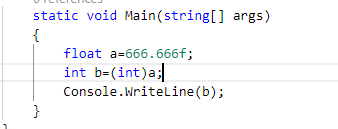– – –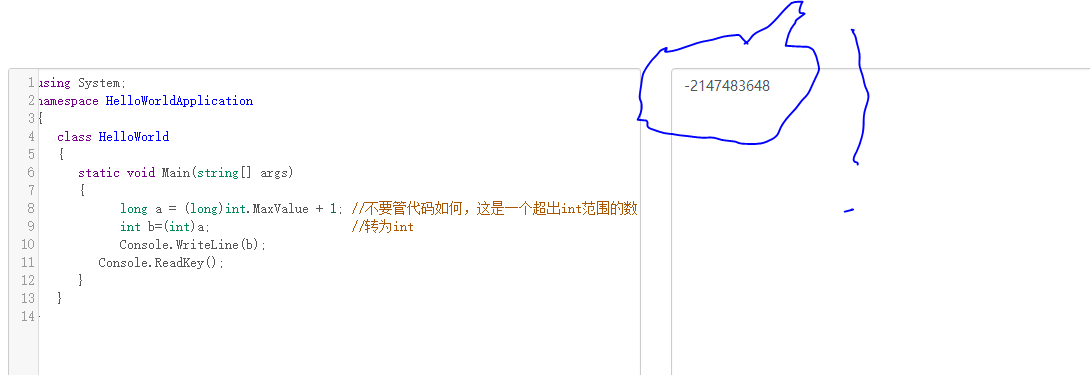char 可以直接跟 int 或 long 使用。

```            int a ='1' + '3';  //小转大
char b = (char)a;  //大转小```
```            int c = 'a' + '5';　　//char可以直接转为 int
```

```
```

注意上下例子的不同 ，int 直接转为char，会报错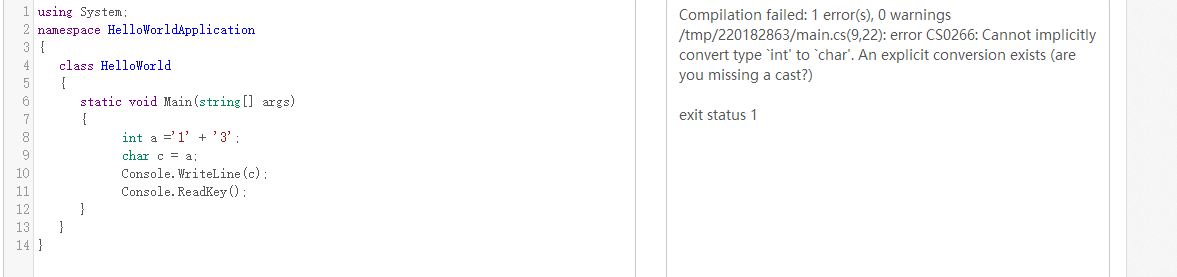([type]) 方法是无法在值类型、引用类型( string 类型)间转换的。

int.Parse()

float.Parse()

… …

```        string str = "666";
long i = int.Parse(str);
Console.WriteLine(\$"{i},{i.GetType()}");

(图如)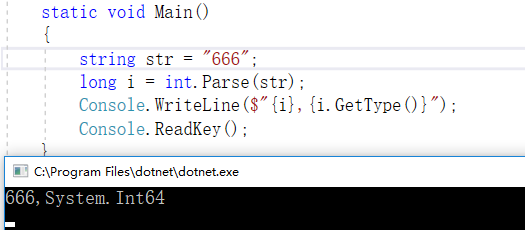Parse() 是专为 string 类型转成 值类型 提供功能的！

Parse() 是为符合数字格式的 string 类型进行转换！

Parse() 是将符合数字格式的字符串转为数字，也就是说，

• ( )括号内的必须为字符串 ！
• 字符串的内容必须为数字 ！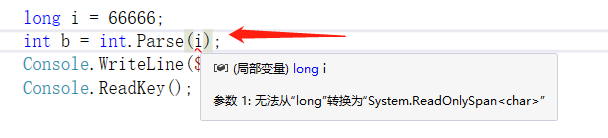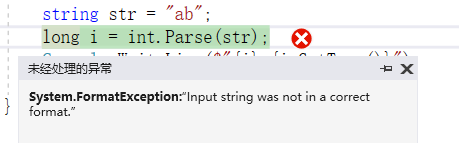int.TryParse()

float.TryParse()

… …

.TryParse() 与 .Parse()  相似，但是使用形式不太一样，

`[类型].TryParse(要更改的字符串,out 存储到哪个变量);　`

```        string str = "666";
int i;
bool b = int.TryParse(str,out i);　　　　//接收转换结果

string str = "666";
int i;
int.TryParse(str,out i);　　　　//不接收转换结果
```

如果你不知道 out 的使用，请查阅 C#中的out参数/ref参数/params可变参数

System.Convert.ToSingle();
System.Convert.ToInt32();
System.Convert.ToDouble();

… …

System.Convert  允许将一种类型转为另一种类型。

```        string str = "666";
int a= System.Convert.ToInt32(str);        //正确

string str = "666.66";
int a= System.Convert.ToInt32(str);        //报错

int a = 66666;
bool c = System.Convert.ToBoolean(a);        //正确

string str = "666.66";
bool c = System.Convert.ToBoolean(str);        //报错

```

System.Convert 在转换是也需要注意 格式 才能转换成功。

同样，此方式也会发生精确度、数字大小改变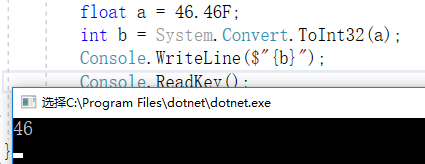第五，System.Convert

.Tostring()

ToString()使用方法最为简单，请留意下列例子的红色背景部分

```using System;
using System.IO;

public class Test
{

static void Main()
{
int a = 666;
string aa = a.ToString();
Console.WriteLine(\$"{aa}");

float b = 666.666F;
string bb = b.ToString();
Console.WriteLine(\$"{bb}");

DateTime c = DateTime.Now;
string cc = c.ToString();
Console.WriteLine(\$"{cc}");

FileInfo d = new FileInfo("E:\\test.txt");
string dd = d.ToString();
Console.WriteLine(\$"{dd}");

string ee;
try
{
int x = 1;
int y = 0;
int e = x / y;
}
catch (Exception ex)
{
ee = ex.ToString();
Console.WriteLine(\$"{ee}");
}

}

}```

```public string ToString(string format, IFormatProvider provider);
public string ToString(string format);
public string ToString(IFormatProvider provider);
public override string ToString();```

```using System;
using System.IO;

public class Test
{

static void Main()
{
int a = 666;
string aa = a.ToString();
Console.WriteLine(\$"{aa}");

float b = 666.666F;
string bb = b.ToString();
Console.WriteLine(\$"{bb}");

DateTime c = DateTime.Now;
string cc = c.ToString();
Console.WriteLine(\$"{cc}");

FileInfo d = new FileInfo("E:\\test.txt");
string dd = d.ToString();
Console.WriteLine(\$"{dd}");

string ee;
try
{
int x = 1;
int y = 0;
int e = x / y;
}
catch (Exception ex)
{
ee = ex.ToString();
Console.WriteLine(\$"{ee}");
}

1.venlafaxine price说道：